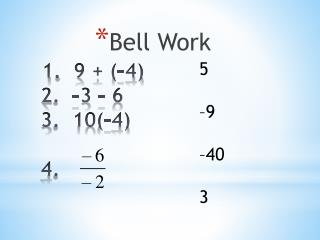DownloadDownload Presentation1. 9 + (–4) 2. –3 – 6 3. 10(–4) 4.

# 1. 9 + (–4) 2. –3 – 6 3. 10(–4) 4.

Download Presentation## 1. 9 + (–4) 2. –3 – 6 3. 10(–4) 4.

- - - - - - - - - - - - - - - - - - - - - - - - - - - E N D - - - - - - - - - - - - - - - - - - - - - - - - - - -
##### Presentation Transcript

1. Bell Work 5 –9 –40 3 1. 9 + (–4)2. –3 – 63. 10(–4)4.

2. One step equations

3. An equation is like a balance scale because it shows that two quantities are equal. What you do to one side of the equation must also be done to the other side to keep it balanced. ONE STEP EQUATIONS

4. One way to solve an equation is to use inverse operations. • An inverse operation is an operation that “undoes” • another operation. • Addition and subtraction are inverse operations. • Multiplication and Division are inverse operations. • *Performing the same operation on each side of an • equation results in a new equation that has the same solution as the original equation.

5. To solve one step equations, you need to ask three questions about the equation: • What is the variable? • What operation is performed on the variable? ONE STEP EQUATIONS • What is the inverse operation? (The one that will undo what is being done to the variable)

6. Example 1 Solve x + 4 = 12 What is the variable? The variable is x. What operation is being performed on the variable? Addition. What is the inverse operation (the one that will undo what is being done to the variable)? Subtraction. Using the subtraction property of equality, subtract 4 from both sides of the equation. The subtraction property of equality tells us to subtract the same thing on both sides to keep the equation equal. ONE STEP EQUATIONS x + 4 = 12 - 4 - 4 x = 8

7. Example 2 Solve y - 7 = -13 What is the variable? The variable is y. What operation is being performed on the variable? Subtraction. What is the inverse operation (the one that will undo what is being done to the variable)? Addition. Using the addition property of equality, add 7 to both sides of the equation. The addition property of equality tells us to add the same thing on both sides to keep the equation equal. ONE STEP EQUATIONS y - 7 = -13 + 7 + 7 y = -6

8. Example 3 Solve –6a = 12 What is the variable? The variable is a. What operation is being performed on the variable? Multiplication. What is the inverse operation (the one that will undo what is being done to the variable)? Division Using the division property of equality, divide both sides of the equation by –6. ONE STEP EQUATIONS The division property of equality tells us to divide the same thing on both sides to keep the equation equal. –6a = 12 -6 -6 a = -2

9. Example 4 Solve -10 = What is the variable? The variable is b. What operation is being performed on the variable? Division. What is the inverse operation (the one that will undo what is being done to the variable)? Multiplication Using the multiplication property of equality, multiply both sides of the equation by 2. The multiplication property of equality tells us to multiply the same thing on both sides to keep the equation equal. ONE STEP EQUATIONS = -10 2 • = -10 • 2 b = -20

10. 6 –6 = 3 m Remember! Once you have solved an equation, it is a good idea to check your answer. To check your answer, substitute your answer for the variable in the original equation. Solve: m + 6 = 9 m + 6 = 9 Since 6 is added to m, subtract 6 from both sides to undo the addition.

11. You try: -9 = y – 12 -9 + 12 = y – 12 + 12 y = 3 Check to see if you are correctIf y =3 is the solution…-9 = y – 12-9 = 3 – 12-9 = -9 solution checks!

12. You try: -3x = 45 -3x ÷ -3 = 45÷ -3 X = -15 Check to see if you are correct… -3x = 45 -3(-15) = 45 45 = 45 Yes!

13. You try: c ÷ 2 = 13 2 x c = 13 x 2 c = 26 Check to see if you are correct… Is 26 is the solution? C÷2 = 13 26÷ 2 = 13 13 = 13 Yes it checks!

14. You try: 1. 3x = – 9 X = -3 -5x = -40 X = 8

15. X - 7 = -1 -X = 9 10 = X + 3 Answers 6 -9 7 24 Try these on your paper.

16. Practice: Worksheet